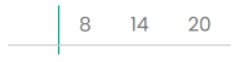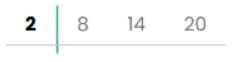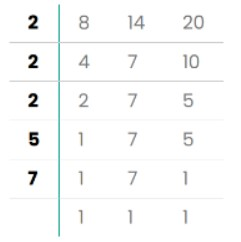# LCD Calculator

To calculate the lowest common divisor (LCD), enter the comma-separated fractions in the input box and hit the calculate button using this LCD calculator.

Give Us Feedback

Use the LCD math calculator on fractions to find their least common denominator. This tool finds the equivalent fraction for each input.

If you want to see the steps of the process then you can find them at the bottom of the LCD calculator. It can find the LCD of mixed numbers, improper proper fractions as well as integers.

## How to use the LCD calculator?

The steps to use this LCD solver are given below.

1. Enter the fractions
2. Click calculate.
3. The LCD will be displayed.

## What is LCD?

In maths, LCD is a similar thing to LCM

It is the abbreviation of the Lowest Common Denominator. A number that is a common multiple of every denominator of the question.

The denominator is the value written under the bar in a fraction. The calculated number is then used to make equivalent fractions to add, subtract, or compare fractions.

## Methods to find LCD:

There are three famous methods to find the LCD.

## LCD formula:

There is one formula to find the least common denominator using the GCF.

LCD(a,b) = |a*b| / GCF (a,b)

As it can be seen, this formula works for only two values. If there is a third value c then the LCD of all three is found by putting the previous LCD and the last value in the formula.

## How to find LCD?

Any of the above-mentioned methods can be used to find the LCD. We recommend the LCD calculator for this purpose for time-saving.

You can learn about prime factorization in the GCF calculator. Here we will discuss the other three methods.

### List of multiples:

In this method, you will list all of the multiples of a number, denominator in this case. Then choose the lowest common factor. This is the LCM or LCD.

Example:

Find the LCD of 6/20, 4 7/8, and 9/14.

Solution:

1. Write every number in fraction form.
= 4 7/8
= (8*4 + 7)/8
= 39/8
2. Separate the denominators.
20, 8, 14
3. List their multiples.
20 :  20, 40, 60, 80, 100, 120, 140, 160, 180, 200, 220, 240, 260, 280, 300, 320.
8 : 8 16 24 32 40 48 56 64 72 80 88 96 104 112 120 128 136 144 152 160 168 176 184 192 200 208 216 224 232 240 248 256 264 272 280 288 296.
14: 14, 28, 42, 56, 70, 84, 98, 112, 126, 140, 154, 168, 182, 196, 210, 224, 238, 252, 266, 280, 294, 308.
As you can see 280 is the first and the lowest common multiple of  10, 8, and 14. It is LCD.
4. Form the equivalent fractions. For this find the number which on multiplying with the denominator will give LCD.

6/20 = ?/280  (you can use the ratio calculator)
6/20 * 14/14 = 84/280

39/8 = ?/280
39/8 * 35/35 = 1365 /280

9/14 = ?/280
9/14 * 20/20 = 180/280

All of these fractions can be simplified to one base fraction.

### Division method:

Write all the denominators in the division ladder. And solve by the least common factor.

Example:
Solve the previous question by the division method for LCD.

Solution:

Skipping the first step of writing in the form of fractions.

1. Write the denominators.2. Divide by the smallest factor.3. Keep dividing until no more division is possible.4. Divide the factors (bold digits in the image) to find the LCD.

= 2 * 2 * 2 * 5 * 7
= 280

### Through GCF:

The last method is to use the GCF to find the least common divisor. The vertical bars in the numerator of the formula mean the absolute value of the product.

Example:
Find the LCD of 5/8, 1 1/12, 4.

Solution:
Step 1: Make each entry a fraction.

1 1/12 = (12*1 + 1)/ 12 = 13/12

And 4 can be written as 4/1.

Step 2: Separate the denominators.

= 8, 12, 1

Step 3: Find the GCF of the first two fractions.

The GCF of the denominators 12 and 8 is 4.

Step 4: Use the formula.

= |8 * 12| / 4
= 24

Step 5: Now, repeat the process for the last fraction.

GCF of 24 and 1 is 24.
= (24 * 1) / GCF (24, 1)
= 24/1
= 24

So the LCD of the 5/8, 1 1/12, 4 is 24.

### Math Tools